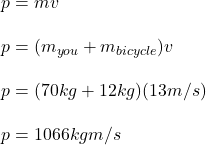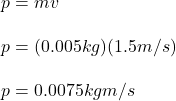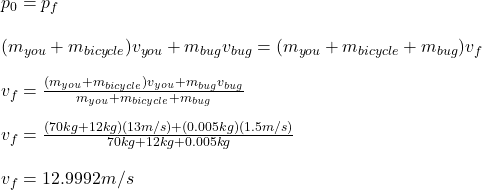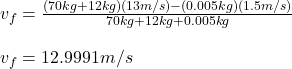## You are coasting on your 12-kg bicycle at 13 m/s and a 5.0-g bug splatters on your helmet. The bug was initially moving at 1.5 m/s in the sa

Question

You are coasting on your 12-kg bicycle at 13 m/s and a 5.0-g bug splatters on your helmet. The bug was initially moving at 1.5 m/s in the same direction as you. If your mass is 70 kg, answer the following questions:a.What is the initial momentum of you plus your bicycle? b.What is the intial momentum of the bug? c.What is your change in velocity due to the collision the bug? d.What would the change in velocity have been if the bug were traveling in the opposite direction?

in progress 0
6 months 2021-08-02T07:31:46+00:00 2 Answers 12 views 0

a. The initial momentum of you and your bicycle is 1066 kgm/s.

b. The initial momentum of the bug is 0.0075 kgm/s.

c. The change in velocity due to the collision with the bug is -0.0008 m/s.

d. If the bug were travelling in the opposite direction, the change in velocity due to the collision would have been -0.0009 m/s.

Explanation:

The initial momentum of you and your bicycle can be easily calculated using the definition of momentum:So the initial momentum of you plus your bicycle is 1066 kgm/s (a).

The initial momentum of the bug can be obtained in the same way:Then the initial momentum of the bug is 0.0075 kgm/s (b).

Now, since the mass of the bug is much less than your mass, we can think of this as a perfectly inelastic collision. This means that, after the collision, the velocity of you, the bicycle and the bug is the same. From the conservation of linear momentum, we have:As your initial velocity was 13m/s, the change in velocity is of -0.0008 m/s (c).

If the bug were travelling in the opposite direction, its initial velocity would have been negative. So:So, in this case the change in velocity is of -0.0009 m/s (d).

Note that the bug is so small that the change in velocity is negligible in most cases. That’s why we don’t notice when we hit a bug when riding bicycle.

a) Pi,c = 1066 kgm/s

b) Pi,b = 0.0075 kgm/s

c) ΔV = – 0.0007 m/s

d) ΔV = – 0.0008 m/s

Explanation:

Given:-

– The mass of the bicycle, mc = 12 kg

– The mass of passenger, mp = 70 kg

– The mass of the bug, mb = 5.0 g

– The initial speed of the bicycle, vpi = 13 m/s

– The initial speed of the bug, vbi = 1.5 m/s

Find:-

a.What is the initial momentum of you plus your bicycle?

b.What is the initial momentum of the bug?

c.What is your change in velocity due to the collision the bug?

d.What would the change in velocity have been if the bug were traveling in the opposite direction?

Solution:-

– First we will set our one dimensional coordinate system, taking right to be positive in the direction of bicycle.

– The initial linear momentum (Pi,c) of the passenger and the bicycle would be:

Pi,c = vpi* ( mc + mp)

Pi,c = 13* ( 12+ 70 )

Pi,c = 1066 kgm/s

– The initial linear momentum (Pi,b) of the bug would be:

Pi,b = vbi*mb

Pi,b = 0.005*1.5

Pi,b = 0.0075 kgm/s

– We will consider the bicycle, the passenger and the bug as a system in isolation on which no external unbalanced forces are acting. This validates the use of linear conservation of momentum.

– The bicycle, passenger and bug all travel in the (+x) direction after the bug splatters on the helmet.

Pi = Pf

Pi,c + Pi,b = V*(mb + mc + mp)

Where,    V : The velocity of the (bicycle, passenger and bug) after collision.

1066 + 0.0075 = V*( 0.005 + 12 + 70 )

V = 1066.0075 / 82.005

V = 12.9993 m/s

The change in velocity is Δv = 13 – 12.9993 =  – 0.00070 m/s

– If the bug travels in the opposite direction then the sign of the initial momentum of the bug changes from (+) to (-).

– We will apply the linear conservation of momentum similarly.

Pi = Pf

Pi,c + Pi,b = V*(mb + mc + mp)

1066 – 0.0075 = V*( 0.005 + 12 + 70 )

V = 1065.9925 / 82.005

V = 12.99911 m/s

The change in velocity is Δv = 13 – 12.99911 =  -0.00088 m/s